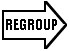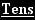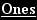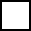Create a new printableMath Worksheets

Sample - Click above to make a new math worksheet (PDF).
 Name _____________________________Date ___________________
Count and then write the tens and ones.
 1 * This is a pre-made sheet.Use the link at the top of the page for a printable page.

Regroup the tens and ones.
2.
 1   ten    11   ones___ tens  ___ one
3.
 2   tens    13   ones___ tens  ___ ones
4.
 5   tens    19   ones___ tens  ___ ones

Add the tens and ones without regrouping. Do you need to regroup? Write the sum.
5.
88  +  6  =

8   tens    14   ones
Do you need to regroup?
 Yes No
What is the sum?

______
6.
47  +  2  =

___ tens  ___ ones
Do you need to regroup?
 Yes No
What is the sum?

______
7.
29  +  6  =

___ tens  ___ ones
Do you need to regroup?
 Yes No
What is the sum?

______

 Key #2
 aKey #2
8.2 3 + 8
9.4 5 + 3
10.3 1 + 9
11.4 4 + 7

12.7 + 2 8
13.1 7 + 4
14.1 + 3 7
15.2 7 + 3

16.3 2 + 1 9
17.5 3 + 2 9
18.7 5 + 1 5
19.4 5 + 4 0

Solve.
20.
Tyler's puzzle has 26 pieces. Joseph's puzzle has 58 pieces. How many pieces do the puzzles have in all?

_____ pieces2 6 + 5 8